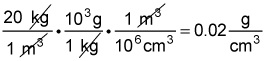##### Chemistry Workbook For Dummies with Online Practice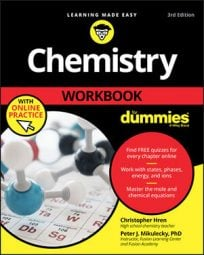Chemists aren’t satisfied with measuring length, mass, temperature, and time alone. On the contrary, chemistry often deals in calculated quantities. These kinds of quantities are expressed with derived units, which are built from combinations of base units.

Here are some examples:

• Area (for example, catalytic surface).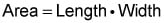and area has units of length squared (square meters, or m2, for example).

• Volume (of a reaction vessel, for example). You calculate volume by using the familiar formula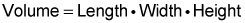Because length, width, and height are all length units, you end up with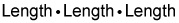or a length cubed (for example, cubic meters, or m³).

• Density (of an unidentified substance). Density, arguably the most important derived unit to a chemist, is built by using the basic formula Density = Mass / Volume.
• Pressure (of gaseous reactants, for example): Pressure units are derived using the formula Pressure = Force / Area.

The SI units for force and area are newtons (N) and square meters (m²), so the SI unit of pressure, the pascal (Pa), can be expressed as N/m².

Let’s try an example. A physicist measures the density of a substance to be 20 kg/m³. His chemist colleague, appalled with the excessively large units, decides to change the units of the measurement to the more familiar grams per cubic centimeter. What is the new expression of the density?

A kilogram contains 1,000 (10³) grams, so 20 kg equals 20,000 g. Well, 100 cm = 1 m; therefore, (100 cm)³= (1 m)³. In other words, there are 100³ (or 106) cubic centimeters in 1 cubic meter. Doing the division gives you 0.02 g/cm³. You can write out the conversion as follows: